Vocabulary
Vocabulary Part 2
Angle Relationships
Angle Relationships Part 2
100

Any line that intersects two or more coplanar lines is known as a what?What is a transversal

100

If two parallel lines are cut by a transversal, these angles are on the inside of the two parallel lines and on the same side of the transversal.

What are Same Side Interior Angles (Consecutive Interior)

100

Two angles are congruent. The measure of the first angles is 42 degrees, what is the measure of the second angle

What is 42

100

If a pair of adjacent angles formed by intersecting line are linear pairs, what is their relationship

What is supplementary

200

Two angles whose measures add up to 180 degrees

What are supplementary angles

200

Two angles whose measures add up to 90o

What are Complementary Angles.

200

What is the relationship between angles 1 and 2?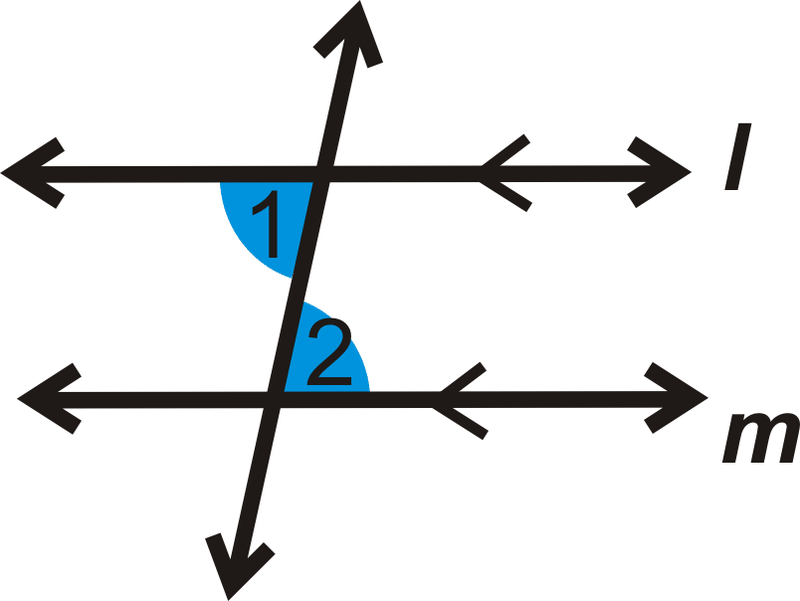What are Alternate Interior Angles.

200

corresponding angles are...

What is congruent

300

When 2 parallel lines are both intersected by a transversal, these angles occupy the same position on the top and bottom parallel lines.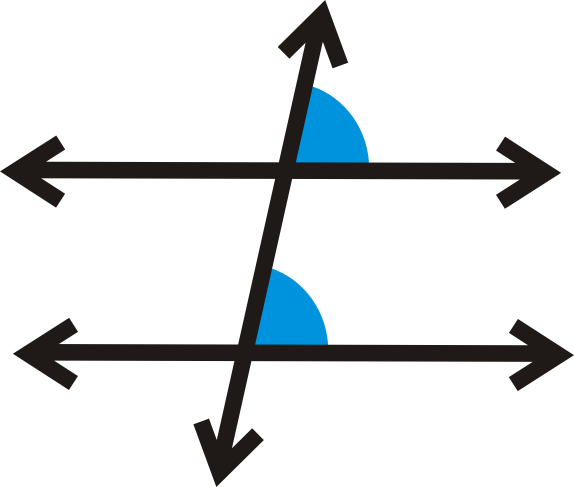What are Corresponding Angles.

300

A pair of adjacent angles formed by intersecting lines.

What are Linear Pair Angles.

300

If the measure of angle 1 is 112, what is the measure of angle 2?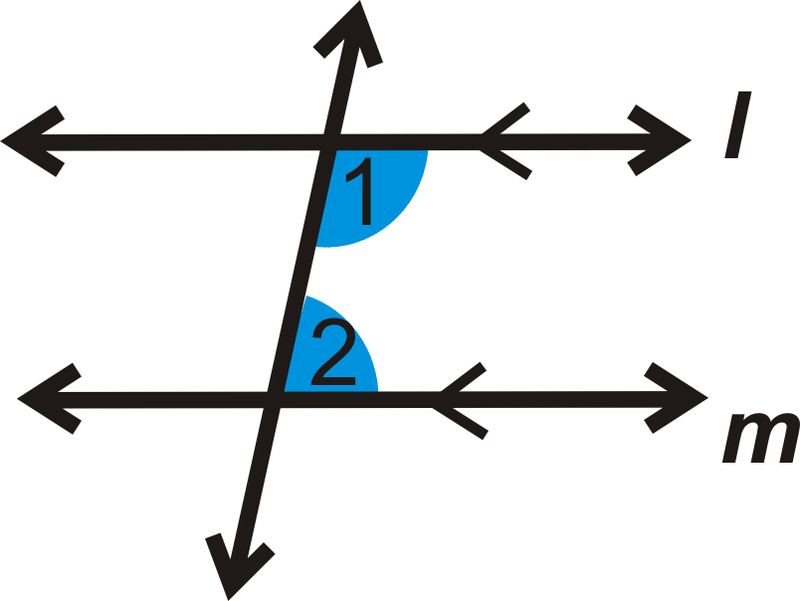What is 68.

300

same side interior angles are

What is supplementary

400

Congruent angles formed by two intersecting linesWhat are Vertical Angles.

400

When 2 parallel lines are both intersected by a transversal these angles are outside of the two parallel lines and on opposite sides of the transversal.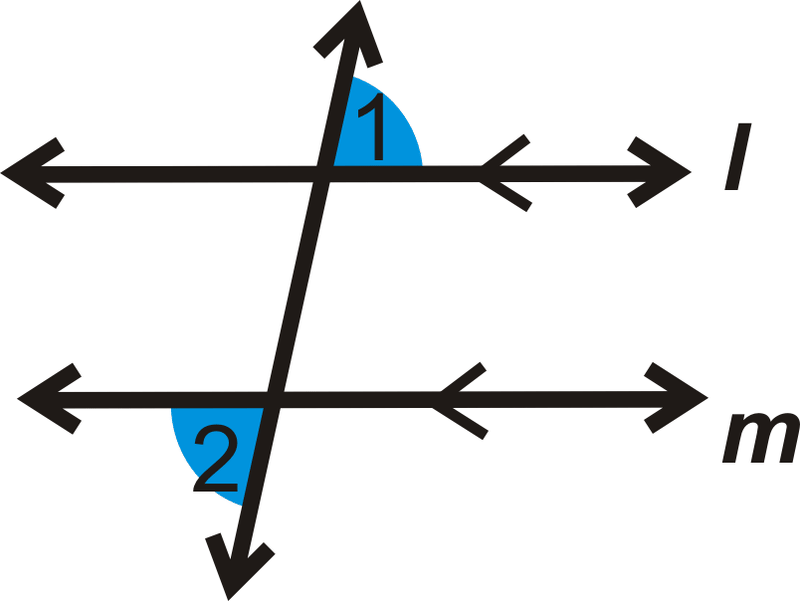Alternate exterior angles
400

What is the relationship between angles 1 and 3?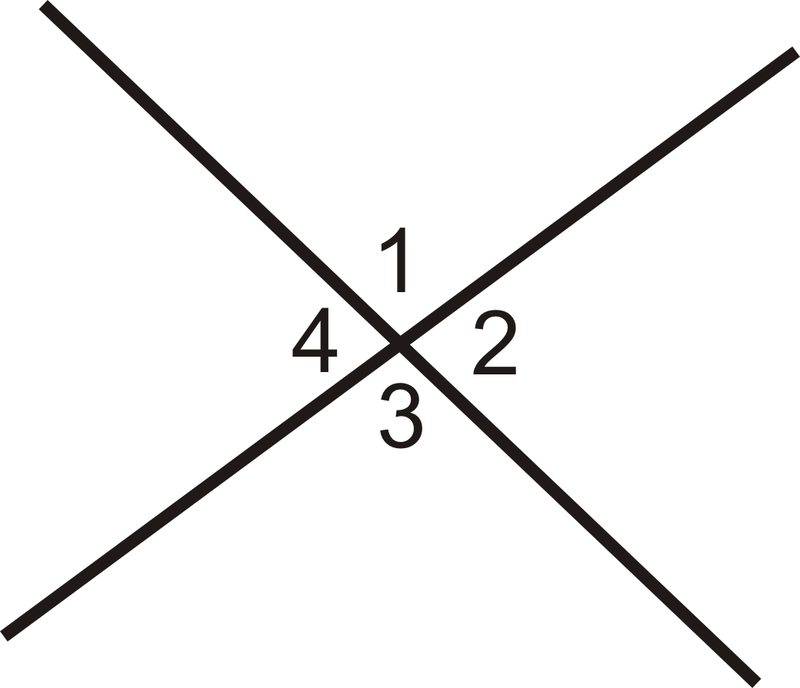What are Vertical Angles.

400

If the measure of angle b is 30 degrees , what is the measure of angle f?What is 30.

500

When 2 parallel lines are both intersected by a transversal these angles are inside of the two parallel lines and on opposite sides of the transversal.What are Alternate Interior Angles.

500

Coplanar lines that do not intersect.

What are parallel lines.

500

If the measure of angle a is 37 degrees, what is the measure of angle h? What is the angle relationshipWhat is 37, Alternate Exterior

500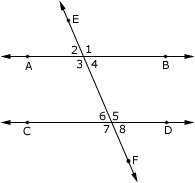Given that angle 8 is 70 degrees, what is the measure of angle 5?  What kind of relationship do these angles have?

5 is 110 degrees, linear pair

Click to zoom